# A survey of fault prediction using machine learning algorithms

26 de Feb de 2012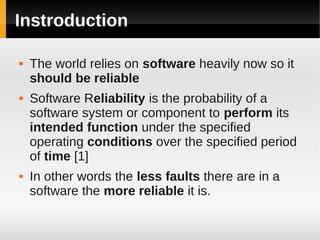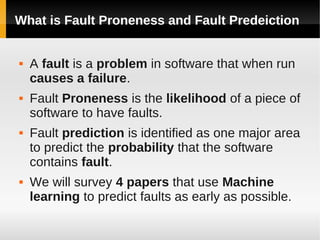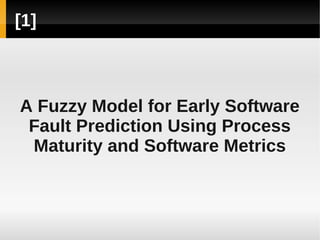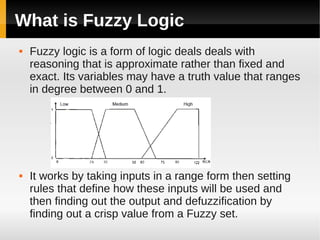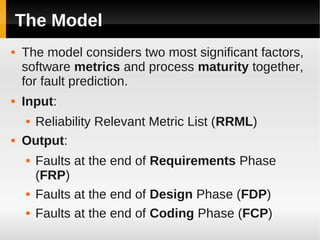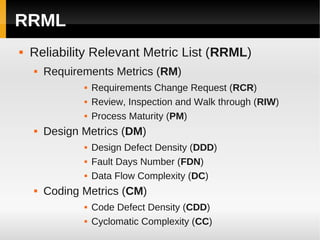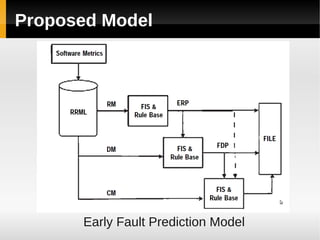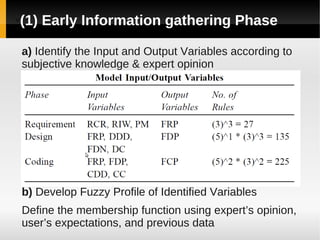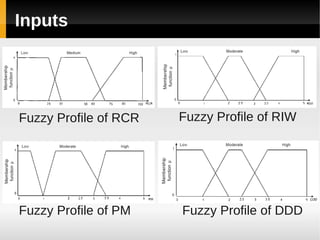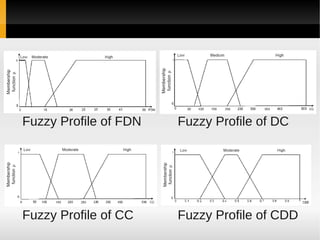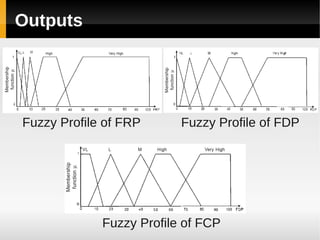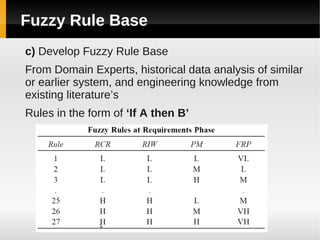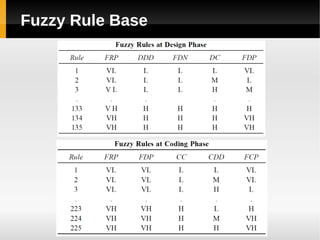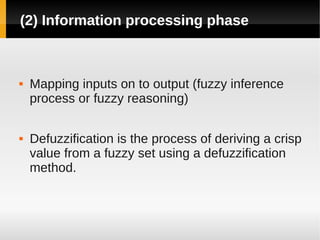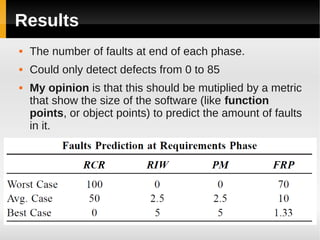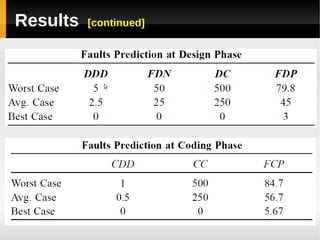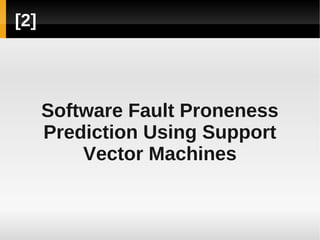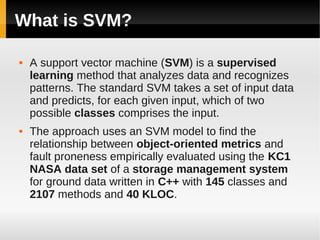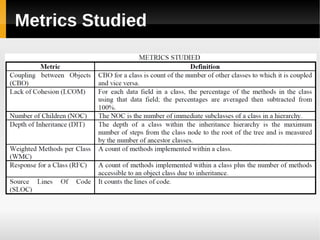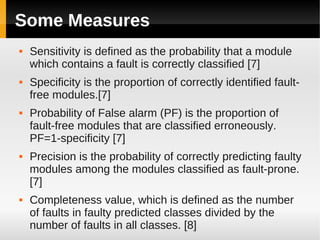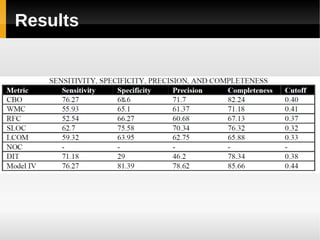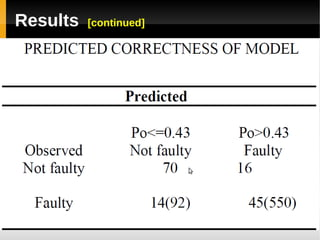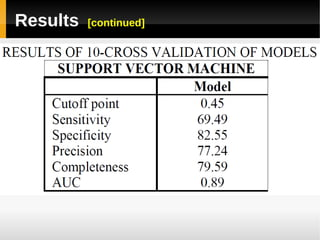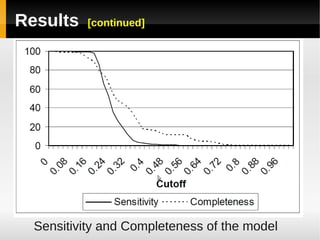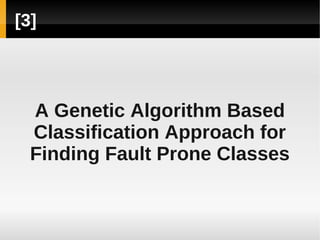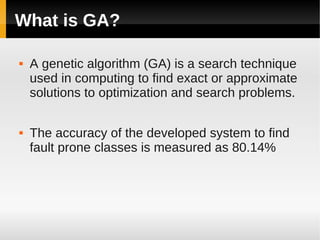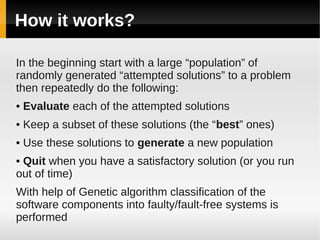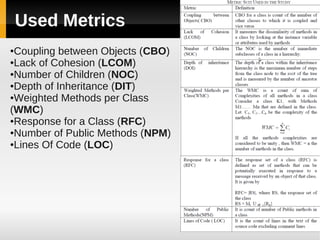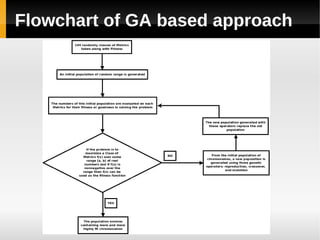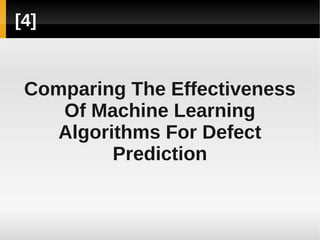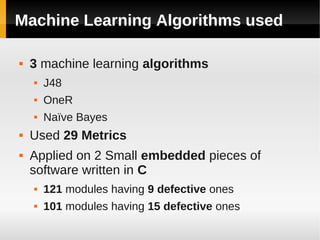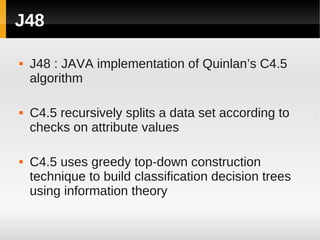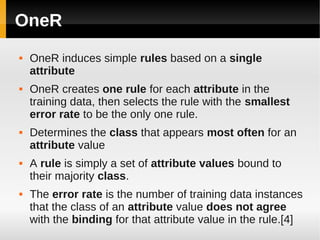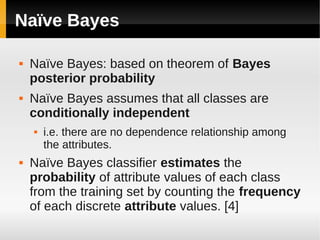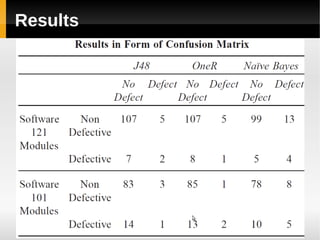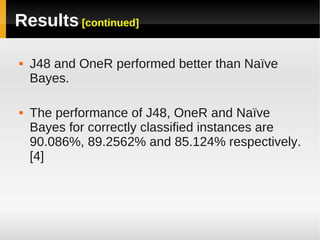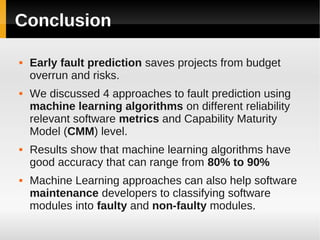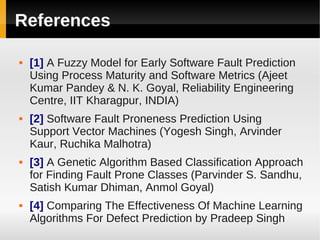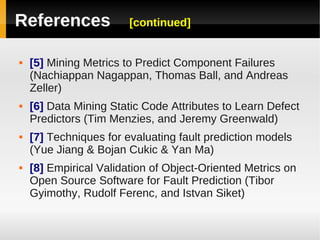1 de 41

### A survey of fault prediction using machine learning algorithms

• 1. A Survey Of Fault Prediction Using Machine Learning Algorithms Presented by: Ahmed Magdy Ezzeldin
• 2. Instroduction  The world relies on software heavily now so it should be reliable  Software Reliability is the probability of a software system or component to perform its intended function under the specified operating conditions over the specified period of time   In other words the less faults there are in a software the more reliable it is.
• 3. What is Fault Proneness and Fault Predeiction  A fault is a problem in software that when run causes a failure.  Fault Proneness is the likelihood of a piece of software to have faults.  Fault prediction is identified as one major area to predict the probability that the software contains fault.  We will survey 4 papers that use Machine learning to predict faults as early as possible.
• 4.  A Fuzzy Model for Early Software Fault Prediction Using Process Maturity and Software Metrics
• 5. What is Fuzzy Logic  Fuzzy logic is a form of logic deals deals with reasoning that is approximate rather than fixed and exact. Its variables may have a truth value that ranges in degree between 0 and 1.  It works by taking inputs in a range form then setting rules that define how these inputs will be used and then finding out the output and defuzzification by finding out a crisp value from a Fuzzy set.
• 6. The Model  The model considers two most significant factors, software metrics and process maturity together, for fault prediction.  Input: Reliability Relevant Metric List (RRML)   Output:  Faults at the end of Requirements Phase (FRP)  Faults at the end of Design Phase (FDP)  Faults at the end of Coding Phase (FCP)
• 7. RRML  Reliability Relevant Metric List (RRML)  Requirements Metrics (RM)  Requirements Change Request (RCR)  Review, Inspection and Walk through (RIW)  Process Maturity (PM)  Design Metrics (DM)  Design Defect Density (DDD)  Fault Days Number (FDN)  Data Flow Complexity (DC)  Coding Metrics (CM)  Code Defect Density (CDD)  Cyclomatic Complexity (CC)
• 8. Proposed Model Early Fault Prediction Model
• 9. (1) Early Information gathering Phase ● a) Identify the Input and Output Variables according to subjective knowledge & expert opinion b) Develop Fuzzy Profile of Identified Variables Define the membership function using expert’s opinion, user’s expectations, and previous data
• 10. Inputs Fuzzy Profile of RCR Fuzzy Profile of RIW Fuzzy Profile of PM Fuzzy Profile of DDD
• 11. Fuzzy Profile of FDN Fuzzy Profile of DC Fuzzy Profile of CC Fuzzy Profile of CDD
• 12. Outputs Fuzzy Profile of FRP Fuzzy Profile of FDP Fuzzy Profile of FCP
• 13. Fuzzy Rule Base c) Develop Fuzzy Rule Base From Domain Experts, historical data analysis of similar or earlier system, and engineering knowledge from existing literature’s Rules in the form of ‘If A then B’
• 14. Fuzzy Rule Base
• 15. (2) Information processing phase  Mapping inputs on to output (fuzzy inference process or fuzzy reasoning)  Defuzzification is the process of deriving a crisp value from a fuzzy set using a defuzzification method.
• 16. Results  The number of faults at end of each phase.  Could only detect defects from 0 to 85  My opinion is that this should be mutiplied by a metric that show the size of the software (like function points, or object points) to predict the amount of faults in it.
• 17. Results [continued]
• 18.  Software Fault Proneness Prediction Using Support Vector Machines
• 19. What is SVM?  A support vector machine (SVM) is a supervised learning method that analyzes data and recognizes patterns. The standard SVM takes a set of input data and predicts, for each given input, which of two possible classes comprises the input.  The approach uses an SVM model to find the relationship between object-oriented metrics and fault proneness empirically evaluated using the KC1 NASA data set of a storage management system for ground data written in C++ with 145 classes and 2107 methods and 40 KLOC.
• 20. Metrics Studied
• 21. Some Measures  Sensitivity is defined as the probability that a module which contains a fault is correctly classified   Specificity is the proportion of correctly identified fault- free modules.  Probability of False alarm (PF) is the proportion of fault-free modules that are classified erroneously. PF=1-specificity   Precision is the probability of correctly predicting faulty modules among the modules classified as fault-prone.   Completeness value, which is defined as the number of faults in faulty predicted classes divided by the number of faults in all classes. 
• 22. Results
• 23. Results [continued]
• 24. Results [continued]
• 25. Results [continued] Sensitivity and Completeness of the model
• 26.  A Genetic Algorithm Based Classification Approach for Finding Fault Prone Classes
• 27. What is GA?  A genetic algorithm (GA) is a search technique used in computing to find exact or approximate solutions to optimization and search problems.  The accuracy of the developed system to find fault prone classes is measured as 80.14%
• 28. How it works? In the beginning start with a large “population” of randomly generated “attempted solutions” to a problem then repeatedly do the following: • Evaluate each of the attempted solutions • Keep a subset of these solutions (the “best” ones) • Use these solutions to generate a new population • Quit when you have a satisfactory solution (or you run out of time) With help of Genetic algorithm classification of the software components into faulty/fault-free systems is performed
• 29. Used Metrics ●Coupling between Objects (CBO) ●Lack of Cohesion (LCOM) ●Number of Children (NOC) ●Depth of Inheritance (DIT) ●Weighted Methods per Class (WMC) ●Response for a Class (RFC) ●Number of Public Methods (NPM) ●Lines Of Code (LOC)
• 30. Flowchart of GA based approach
• 31.  Comparing The Effectiveness Of Machine Learning Algorithms For Defect Prediction
• 32. Machine Learning Algorithms used  3 machine learning algorithms  J48  OneR  Naïve Bayes  Used 29 Metrics  Applied on 2 Small embedded pieces of software written in C  121 modules having 9 defective ones  101 modules having 15 defective ones
• 33. J48  J48 : JAVA implementation of Quinlan’s C4.5 algorithm  C4.5 recursively splits a data set according to checks on attribute values  C4.5 uses greedy top-down construction technique to build classification decision trees using information theory
• 34. OneR  OneR induces simple rules based on a single attribute  OneR creates one rule for each attribute in the training data, then selects the rule with the smallest error rate to be the only one rule.  Determines the class that appears most often for an attribute value  A rule is simply a set of attribute values bound to their majority class.  The error rate is the number of training data instances that the class of an attribute value does not agree with the binding for that attribute value in the rule.
• 35. Naïve Bayes  Naïve Bayes: based on theorem of Bayes posterior probability  Naïve Bayes assumes that all classes are conditionally independent  i.e. there are no dependence relationship among the attributes.  Naïve Bayes classifier estimates the probability of attribute values of each class from the training set by counting the frequency of each discrete attribute values. 
• 36. Results
• 37. Results [continued]  J48 and OneR performed better than Naïve Bayes.  The performance of J48, OneR and Naïve Bayes for correctly classified instances are 90.086%, 89.2562% and 85.124% respectively. 
• 38. Conclusion  Early fault prediction saves projects from budget overrun and risks.  We discussed 4 approaches to fault prediction using machine learning algorithms on different reliability relevant software metrics and Capability Maturity Model (CMM) level.  Results show that machine learning algorithms have good accuracy that can range from 80% to 90%  Machine Learning approaches can also help software maintenance developers to classifying software modules into faulty and non-faulty modules.
• 39. References   A Fuzzy Model for Early Software Fault Prediction Using Process Maturity and Software Metrics (Ajeet Kumar Pandey & N. K. Goyal, Reliability Engineering Centre, IIT Kharagpur, INDIA)   Software Fault Proneness Prediction Using Support Vector Machines (Yogesh Singh, Arvinder Kaur, Ruchika Malhotra)   A Genetic Algorithm Based Classification Approach for Finding Fault Prone Classes (Parvinder S. Sandhu, Satish Kumar Dhiman, Anmol Goyal)   Comparing The Effectiveness Of Machine Learning Algorithms For Defect Prediction by Pradeep Singh
• 40. References [continued]   Mining Metrics to Predict Component Failures (Nachiappan Nagappan, Thomas Ball, and Andreas Zeller)   Data Mining Static Code Attributes to Learn Defect Predictors (Tim Menzies, and Jeremy Greenwald)   Techniques for evaluating fault prediction models (Yue Jiang & Bojan Cukic & Yan Ma)   Empirical Validation of Object-Oriented Metrics on Open Source Software for Fault Prediction (Tibor Gyimothy, Rudolf Ferenc, and Istvan Siket)
• 41. Thank You Questions?